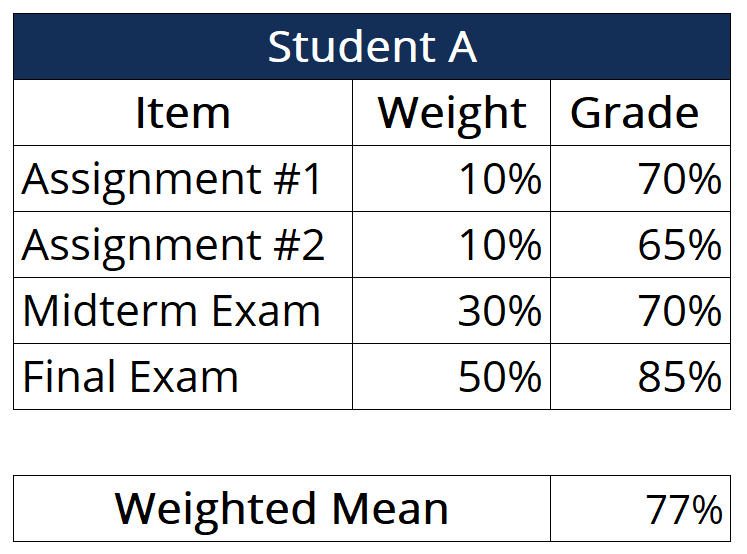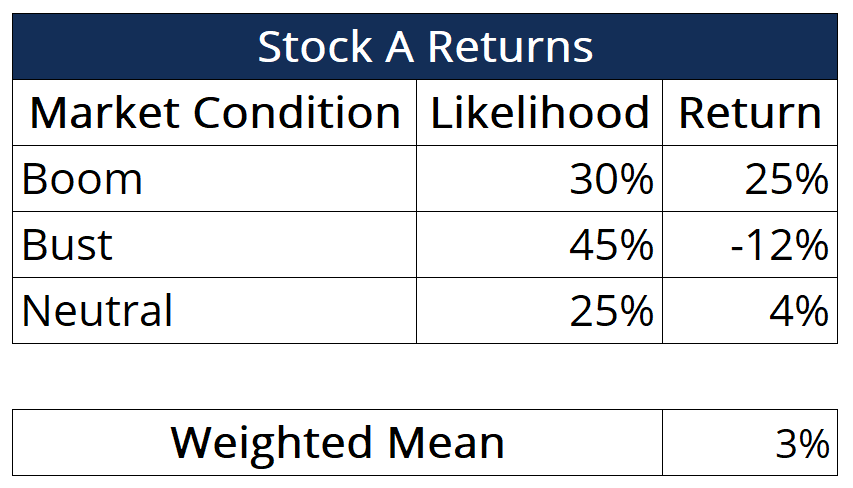# Weighted Mean

Multiplying the weight of a particular event or outcome with its associated quantitative outcome and then summing all the products

## What is the Weighted Mean?

The weighted mean is a type of mean that is calculated by multiplying the weight (or probability) associated with a particular event or outcome with its associated quantitative outcome and then summing all the products together. It is very useful when calculating a theoretically expected outcome where each outcome shows a different probability of occurring, which is the key feature that distinguishes the weighted mean from the arithmetic mean.It is important to note that all the probabilities or weights must be mutually exclusive (i.e., no two events can occur at the same time) and that the total weights and probabilities must add up to 100%.

When calculating an arithmetic mean, we make the assumption that all numbers used in the calculation show an equal probability of occurring or associated weights. Thus, we do not need to account for the differences and can simply sum up the numbers that we are interested in finding the mean of and then dividing the sum by the number of observations.

### Uses of Weighted Means

Weighted means are useful in a wide variety of scenarios. For example, a student may use a weighted in order to calculate his/her percentage grade in a course. In such an example, the student would multiply the weighing of all assessment items in the course (e.g., assignments, exams, projects, etc.) by the respective grade that was obtained in each of the categories. Consider the following student with the following grades:In the example above, we can arrive at the weighted mean by multiplying the weights associated with each assessment item by the grade that the student obtained on each of the items. Then, we can sum the products and arrive at the student’s final grade.

Here, we see that the student is actually able to get a better than expected grade by doing well in the most heavily weighted component of the course: the final. Given the knowledge of the weighing of each assessment element in the course, students can allocate their study time more effectively.

Taking a step back, students will also be better equipped to balance a particular assessment item against other time-consuming activities (e.g., social life, personal hobbies, other courses, etc.) and make decisions that match their personal utility function.

### Expected Returns

In the context of finance, the (weighted) means are used to calculate expected values or returns on certain investments. For instance, suppose that financial analysts observe the behavior of certain securities under different market conditions over a large period of time.

Next, financial analysts can take into account the macroeconomic climate that pervades in the relevant jurisdictions and determine the likelihood of a bull market, as well as the likelihood of a bear market. From the analysis, we can make better investment choices by calculating an expected return figure that will summarize the level of investment returns that we can expect to see.

Consider the following stock, Stock A:Similar to the student grade example, we can calculate the weighted mean (in this case, the expected return) of the stock by summing the products of the probabilities and returns.

While we see that the stock can achieve a high return of 25%, the return will only occur if market conditions are exceptional, which is a case with a fairly low probability. As opposed to such a situation, we see that the expected return under poor market conditions is of comparatively smaller magnitude, but the likelihood of the market doing poorly is far greater than the likelihood of the market doing well.

### More Resources

CFI is the official provider of the Financial Modeling and Valuation Analyst (FMVA)™ certification program, designed to transform anyone into a world-class financial analyst.

To keep learning and developing your knowledge of financial analysis, we highly recommend the additional resources below:

• Basic Statistics Concept for Finance
• Geometric Mean
• Sharpe Ratio Calculator
• Capitalization-Weighted Index

### Financial Analyst Certification

Become a certified Financial Modeling and Valuation Analyst (FMVA)® by completing CFI’s online financial modeling classes and training program!